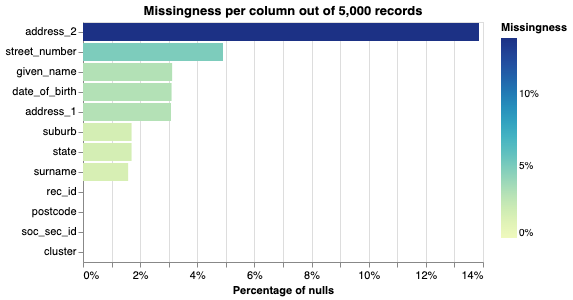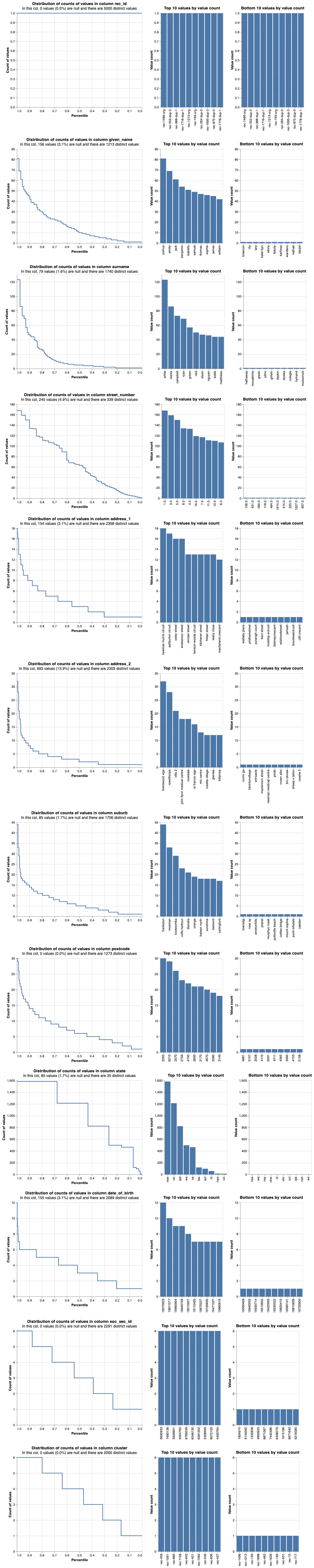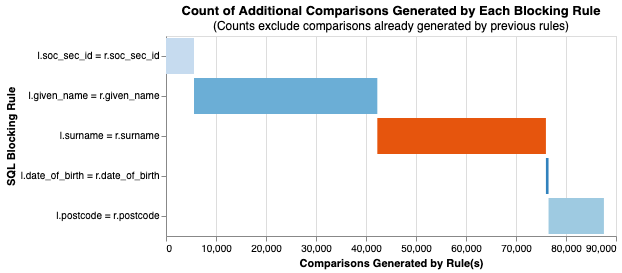# Febrl3 Dedupe

## Deduplicating the febrl3 dataset¶

See A.2 here and here for the source of this data

import pandas as pd
import altair as alt
alt.renderers.enable('mimetype')

df = pd.read_csv("./data/febrl/dataset3.csv", delimiter=", ", dtype={"date_of_birth":str}, engine="python")
df["cluster"] = df["rec_id"].apply(lambda x: "-".join(x.split('-')[:2]))

rec_id given_name surname street_number address_1 address_2 suburb postcode state date_of_birth soc_sec_id cluster
0 rec-1496-org mitchell green 7.0 wallaby place delmar cleveland 2119 sa 19560409 1804974 rec-1496
1 rec-552-dup-3 harley mccarthy 177.0 pridhamstreet milton marsden 3165 nsw 19080419 6089216 rec-552
from splink.duckdb.duckdb_linker import DuckDBLinker

settings = {
"unique_id_column_name": "rec_id",
}


linker.missingness_chart()linker.profile_columns(list(df.columns))potential_blocking_rules = [
"l.soc_sec_id = r.soc_sec_id",
"l.given_name = r.given_name",
"l.surname = r.surname",
"l.date_of_birth = r.date_of_birth",
"l.postcode = r.postcode"
]from splink.duckdb.duckdb_linker import DuckDBLinker

settings = {
"unique_id_column_name": "rec_id",
"blocking_rules_to_generate_predictions": potential_blocking_rules,
"comparisons": [
{
"output_column_name": "Given name",
"comparison_levels": [
cll.null_level("given_name"),
{
"sql_condition": '"given_name_l" = "surname_r"',
"label_for_charts": "Exact match on reversed cols, l to r",
},
cll.distance_function_level(
"given_name", "jaro_winkler_similarity", 0.9
),
cll.distance_function_level(
"given_name", "jaro_winkler_similarity", 0.7
),
{
"sql_condition": "jaro_winkler_similarity(given_name_l, surname_r) > 0.7",
"label_for_charts": "Jar on reversed cols, l to r",
},
cll.else_level(),
],
},
{
"output_column_name": "Surname",
"comparison_levels": [
cll.null_level("surname"),
{
"sql_condition": '"given_name_r" = "surname_l"',
"label_for_charts": "Exact match on reversed cols, r to l",
},
cll.distance_function_level("surname", "jaro_winkler_similarity", 0.9),
cll.distance_function_level("surname", "jaro_winkler_similarity", 0.7),
{
"sql_condition": "jaro_winkler_similarity(given_name_r, surname_l) > 0.7",
"label_for_charts": "Jaro on reversed cols, l to r",
},
cll.else_level(),
],
},
cl.levenshtein_at_thresholds(
),
cl.levenshtein_at_thresholds("soc_sec_id", ),
],
"retain_intermediate_calculation_columns": True,
}


deterministic_rules = [
"l.soc_sec_id = r.soc_sec_id",
"l.given_name = r.given_name and l.surname = r.surname and l.date_of_birth = r.date_of_birth",
"l.given_name = r.surname and l.surname = r.given_name and l.date_of_birth = r.date_of_birth"
]


Probability two random records match is estimated to be  0.000527.
This means that amongst all possible pairwise record comparisons, one in 1,899.32 are expected to match.  With 12,497,500 total possible comparisons, we expect a total of around 6,580.00 matching pairs


linker.estimate_u_using_random_sampling(target_rows=1e6)

----- Estimating u probabilities using random sampling -----

Estimated u probabilities using random sampling

Your model is not yet fully trained. Missing estimates for:
- Given name (no m values are trained).
- Surname (no m values are trained).
- date_of_birth (no m values are trained).
- soc_sec_id (no m values are trained).
- street_number (no m values are trained).
- postcode (no m values are trained).


comparison = linker._settings_obj.comparisons.as_dict()

session_dob = linker.estimate_parameters_using_expectation_maximisation("substr(l.date_of_birth,1,3) = substr(r.date_of_birth,1,3)")


----- Starting EM training session -----

Estimating the m probabilities of the model by blocking on:
substr(l.date_of_birth,1,3) = substr(r.date_of_birth,1,3)

Parameter estimates will be made for the following comparison(s):
- Given name
- Surname
- soc_sec_id
- street_number
- postcode

Parameter estimates cannot be made for the following comparison(s) since they are used in the blocking rules:
- date_of_birth

Iteration 1: Largest change in params was -0.493 in probability_two_random_records_match
Iteration 2: Largest change in params was -0.00611 in the m_probability of soc_sec_id, level All other comparisons
Iteration 3: Largest change in params was -0.000544 in the m_probability of soc_sec_id, level All other comparisons
Iteration 4: Largest change in params was -6.13e-05 in the m_probability of soc_sec_id, level All other comparisons

EM converged after 4 iterations

Your model is not yet fully trained. Missing estimates for:
- date_of_birth (no m values are trained).

----- Starting EM training session -----

Estimating the m probabilities of the model by blocking on:
substr(l.postcode,1,2) = substr(r.postcode,1,2)

Parameter estimates will be made for the following comparison(s):
- Given name
- Surname
- date_of_birth
- soc_sec_id
- street_number

Parameter estimates cannot be made for the following comparison(s) since they are used in the blocking rules:
- postcode

Iteration 1: Largest change in params was -0.234 in probability_two_random_records_match
Iteration 2: Largest change in params was -0.0152 in the m_probability of soc_sec_id, level All other comparisons
Iteration 3: Largest change in params was -0.000974 in the m_probability of soc_sec_id, level All other comparisons


linker.match_weights_chart()

results = linker.predict(threshold_match_probability=0.2)

linker.roc_chart_from_labels_column("cluster")

pred_errors_df = linker.prediction_errors_from_labels_column("cluster").as_pandas_dataframe()
len(pred_errors_df)

records = linker.prediction_errors_from_labels_column("cluster").as_record_dict(limit=10)